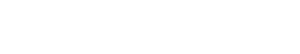# Independent Variable

Independent Variable is used to refer to the input data that is used to train machine learning models. This input data can be in the form of text, images, or other types of data. Independent Variable is also sometimes used outside of the text analytics industry, but it can have different meanings in different contexts. For example, in statistics, an independent variable is a variable that is not affected by other variables.

Identifying independent variables, also known as Predictor variables, is a crucial step in any machine learning or predictive modeling project.

## Single Independent Variable

When there is a single independent variable, it is referred to as a univariate model.

There are two categories of univariate model:

• Categorical independent variable can take on a limited, and usually fixed a number of values, such as male/female or true/false.
• Numerical independent variables can take on any value within a range.

Univariate analysis is used to determine the effect of one independent variable on the dependent variable.

how to plot a univariate model

## Multiple Independent Variables

Multivariate models are used when there is more than one independent variable.

The number of independent variables in a multivariate model can be two (bivariate), three (trivariate), or more (multivariate).

There are two types of multivariate models: linear and nonlinear. Linear models are the most common and easiest to understand. In a linear model, the dependent variable is a linear function of the independent variables. Nonlinear models are more complex but can be more accurate for certain types of data. In a nonlinear model, the dependent variable is a nonlinear function of the independent variables.

An important thing to remember is that, regardless of the type of model you are using, all models are based on a set of assumptions. It is important to check that your data meets these assumptions before you proceed with your analysis.Unlock the power of actionable insights with AI-based natural language processing.